# Fast RGB to Spectrum Conversion for ReflectancesPublished September 26, 2018 by Scott Allen Burns, last updated Oct 3, 2018

Change Log
9/26/18 Initial publication.
9/27/18 To avoid confusion with established colorimetric terminology, the word “primaries” has been changed to “components.”
9/28/18 Added section of computational speed.
9/28/18 Added section on comparing RGBC to measured reflectances of Munsell color chips.
9/30/18 Added the phrase “gamma correction” to “companding” since it is a more commonly used term.
10/3/18 Added section showing WGM blending of RGBC and ILLSS side-by-side.

#### Introduction

In a previous presentation on generating reflectance curves from sRGB triplets, I presented several algorithms for creating a reasonably realistic spectral reflectance curve associated with a given sRGB color. These algorithms require considerable computational effort, which limit their applicability in computationally intensive tasks such as computer graphics rendering via ray-tracing.

I was approached by an interested reader, Brien Dieterle, who suggested that the computational burden could be lessened by using these algorithms to generate just three reflectance curves, associated with sRGB values of [255, 0 0], [0, 255, 0], and [0, 0, 255], and using them to create additional reflectances for other colors by simply computing a weighted sum of these three reflectance curves.

The three reflectance curves produced by the ILLSS algorithm (see ref) corresponding to the sRGB values of (255, 0, 0), (0, 255, 0), and (0, 0, 255), respectively, have the shapes below: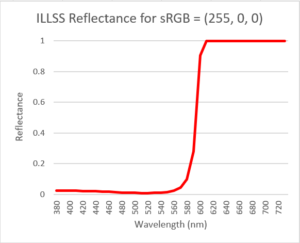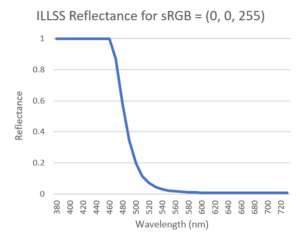To find a reflectance curve for an arbitrary sRGB value, Brien’s method first converts the sRGB to linear rgb (by removing the companding/gamma correction), giving the three rgb values in the range 0 to 1. Then the three component reflectance curves above are multiplied by the corresponding r, g, and b values, and then summed together. For example, for sRGB = (125, 150, 100) the linear rgb is (0.2051, 0.3050, 0.1274), and the weighted sum of the three components becomes: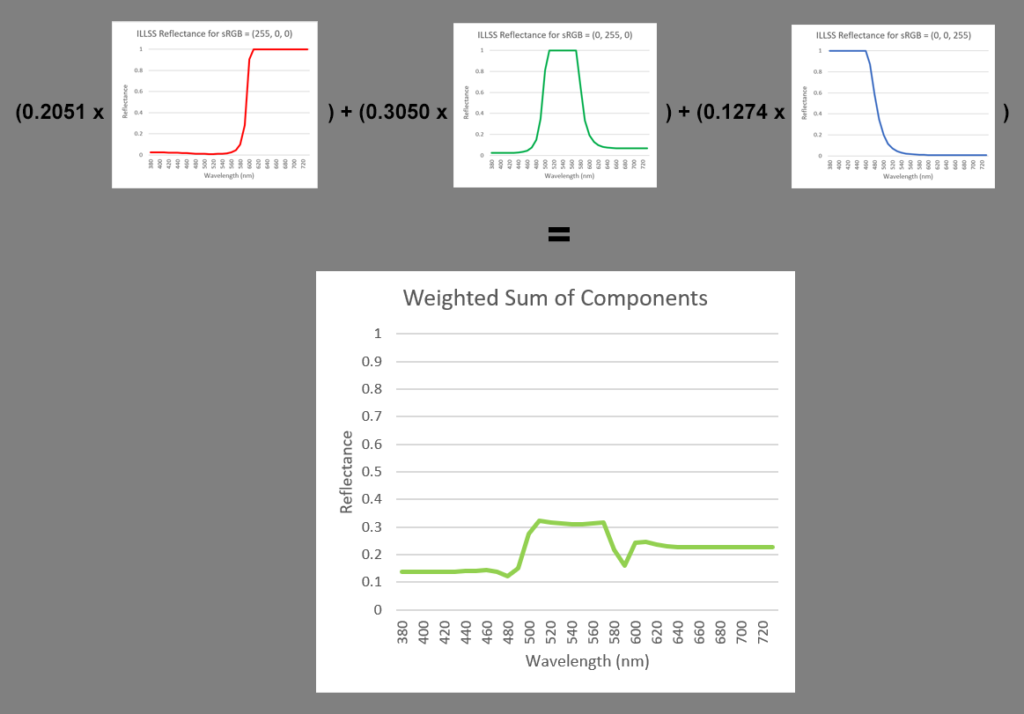It is important to use linear rgb instead of the companded (gamma corrected) sRGB because linear rgb preserves the mathematical property of additivity, and consequently, the rgb values of the weighted sum will match the original rgb values.

sRGB to rgb conversion
The process of removing the companding (gamma correction) from sRGB to give rgb is:
sRGB=sRGB/255; % convert 0-255 range to 0-1 range for i=1:3   if sRGB(i)<0.04045     rgb(i)=sRGB(i)/12.92;   else     rgb(i)=((sRGB(i)+0.055)/1.055)^2.4;   end end

To convert rgb back to sRGB:
 for i=1:3   if rgb(i)<0.0031308     sRGB(i)=12.92*rgb(i);   else     sRGB(i)=1.055*rgb(i)^(1/2.4)-0.055;   end end sRGB=round(255*sRGB); % convert to 0-255

One downside to the weighted sum of components approach is that the resulting reflectance curve is quite jagged. But as Brien pointed out to me, the more worrisome aspect is that the weighted sum might exceed a value of 1 in some regions. In fact, when applying it to sRGB = (255, 255, 255), which is rgb = (1, 1, 1), the weighted sum is simply the sum of the three components: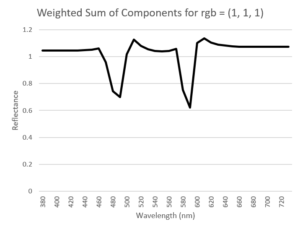Large portions of the composite reflectance curve have values > 1, which can be problematic in many applications.

This brings us to the goal of this presentation. Brien asked me if I could think of some way to design three component spectral distributions so that their weighted sum would never exceed a value of 1. He suggested that perhaps some modification of the optimization approach I used in the previous work could be applied here as well. I thought it sounded like a fun challenge, and I managed to find a solution, as presented in the next section.

#### Three Optimal RGB Components

Recall that the ILLSS (Iterative Log Least Slope Squared) method is based on the solution of this nonlinear program: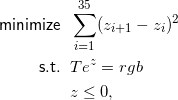where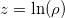and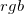is the target linear rgb triplet. The reflectance vector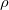has 36 elements, representing reflectance values for wavelengths 380 nm to 730 nm in 10 nm intervals. The objective function is a discretized version of the integral of the square of the the slope of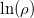. The first constraint set enforces thathas a corresponding rgb value matching the target color.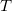is a 3×36 matrix that produces linear rgb (D65 weighted, and not gamma corrected) when premultiplying. The second constraint set enforces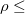1 (note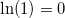). This nonlinear program has 36 variables and 39 constraints.

To adapt it to find three optimal RGB components, the set of variables is expanded to three sets ofvectors, one for each component, named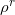,, and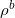. The corresponding log ofvalues are called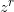,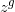,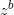. The objective function is expanded to minimize the sum of the three log-slope-squared sums. The constraint set is expanded to make sure,, and, have linear rgb values of (1,0,0), (0,1,0), and (0,0,1), respectively, and that the sum of the threevalues is always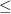1. This gives rise to a nonlinear program with 108 variables and 45 constraints: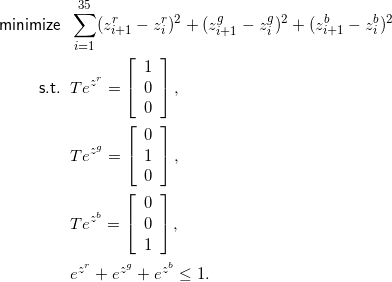In the previous work, the ILLSS method relied on an iterative scheme to identify which of the inequality constraints were active at the solution, and subsequently converted to equality constraints. This was done to make the method more computationally tractable. In this case, the nonlinear program needs to be solved only once, and the three solution vectors (,, and) are then used to generate composite reflectance curves after that. So it is reasonable to pull out the “heavy artillery” to solve the nonlinear program, namely, a general purpose optimization code such as Matlab’s fmincon, or Excel’s Solver add-in.

Here is a Matlab program that solves this optimization. The three solution vectors are available here, and are plotted below: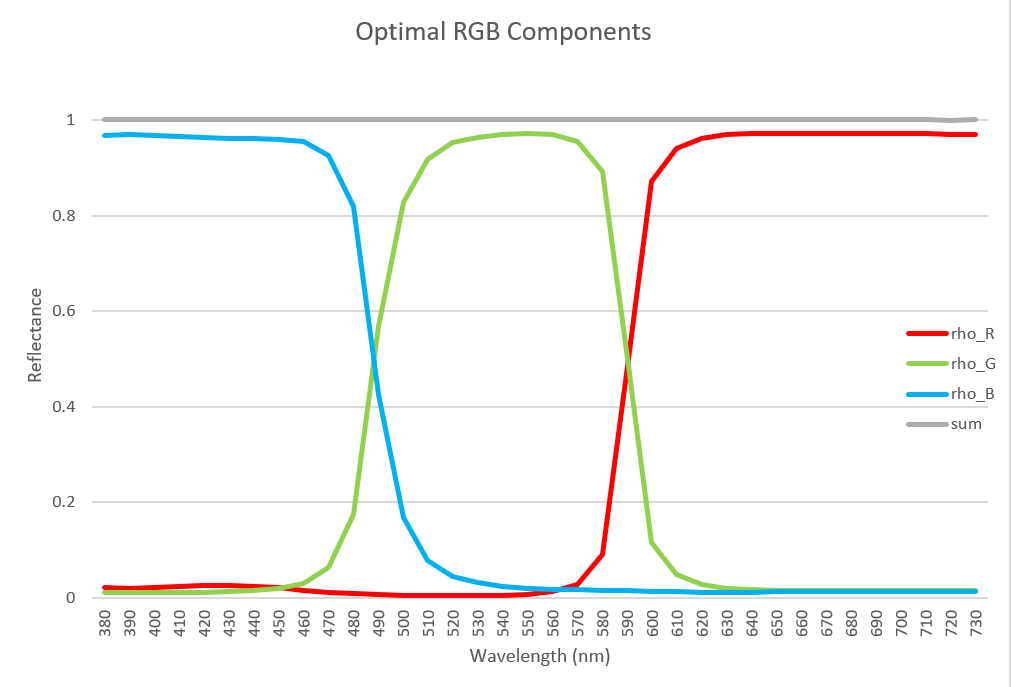The sum of the three components is also plotted as a gray line, and turns out to be equal to 1 everywhere.

In summary, the RGB Components (RGBC) method is:
1) Convert sRGB to linear rgb, where r, g, and b are each in the range 0 to 1 (if you are already working in a linear rgb environment, you can skip this step).
2) Compute the sum of three weighted vectors, rho = r*rho_R + g*rho_G + b*rho_B.
3) Done!

Consider the example presented above, sRGB = (125, 150, 100) and linear rgb = (0.2051, 0.3050, 0.1274). The plot below shows the reflectance obtained from the optimal RGB Components method and from the ILLSS method.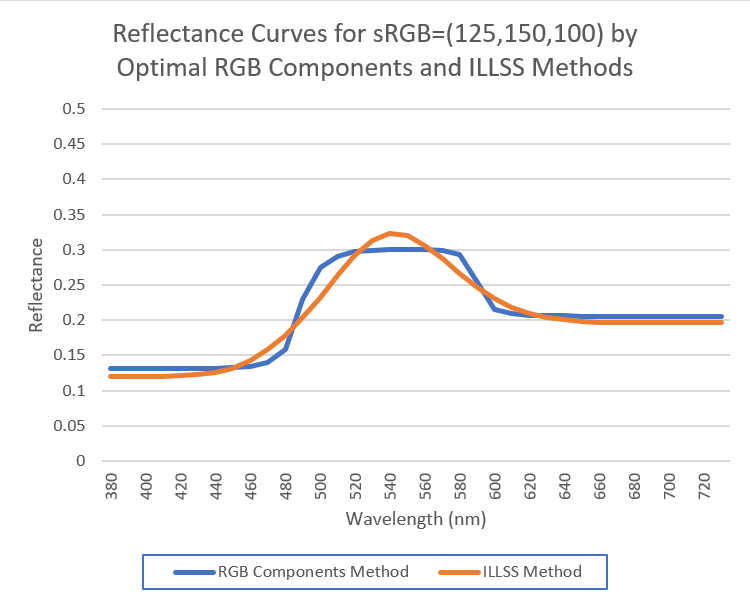The two compare very favorably. As expected, the ILLSS curve is smoother in the sense of less slope squared, but the RGB components curve is also quite smooth. The next sections will look more in depth at comparing the RGB components method to other methods.

#### Computational Efficiency Comparison

The RGB Components (RGBC) method requires only the scaling and addition of three 36-element vectors. This is the same effort as is required for the simple matrix multiplication involved in the LLS (Linear Least Squares) and LSS (Least Slope Squared) methods of the previous presentation. The RGBC method guarantees that the reflectance curve will fall within the 0 to 1 range, a claim neither LLS nor LSS can make. It is also roughly 200 times faster than ILLSS. The only other previous method that guarantees the 0 to 1 result is ILSS (Iterative Least Slope Squared), which is about 10 times slower than RGBC.

#### Comparison to Munsell Color Spectra

This section compares the reflectance curves generated by RGBC to the curves measured spectrophotometrically from all 1296 sRGB-in-gamut 2007 Munsell glossy edition color chips. See the previous publication for more information on this data set. A similarity measure was established, called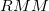,which weights the difference in reflectance curves by the relative sensitivity of the eye to various wavelengths (i.e., via the luminosity curve).

 Name MaxMeanRGBC, RGB Components method 1.39 0.27 ILSS, Iterative Least Slope Squared 1.04 0.16 ILLSS, Iterative Least Log Slope Squared 0.86 0.15

On average, the RGBC results have about twice the deviation from the Munsell measurements as the ILLSS method. Here is a side-by-side comparison of all 1296values, where black is mapped to 1.39 (the largest deviation) and white is mapped to zero (click figures to enlarge):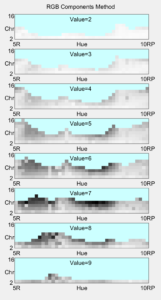Generally speaking, RGBC has the largest deviations from the Munsell measurements in the saturated yellow and blue regions. The two largest deviations are for Munsell colors 2.5B 7/8 and 7.5Y 7/12: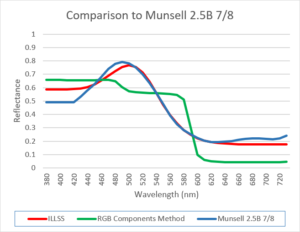The tendency for RGBC to generate broad waveforms actually works to its advantage, in comparison to ILLSS, which tends to have too narrow of peaks in the yellow range of colors:

It is not too surprising that the RGBC results are not as good as other optimization-based methods. There are only three degrees of freedom in the RGBC method (the three weighting factors on the rho_R, rho_G, and rho_B), whereas ILSS and ILLSS have more degrees of freedom available to shape the curves.

#### Subtractive Mixture Comparison

In this section, I compare the subtractive blending using the weighted geometric mean (WGM), as described here. ILLSS is on the left and RGBC is on the right. In each plot, two sRGB colors are blended together, and those blends are also tinted to white and shaded to black (actually a reflectance of 0.02 is used for black, as zero is not an appropriate value for WGM mixture).

Overall, the comparison is very positive. There does appear to be a little hue shifting (Abney effect?) in the blue to white blends in the RGBC method that isn’t present in the ILLSS blends. And there seems to be the most difference between the two methods in the central region of the green-magenta blend, but it is still minor.

#### Acknowledgments

Thanks to Brien Dieterle for coming up with the idea of using ILLSS-generated RGB components to greatly increase the computational efficiency of reflectance curve generation, and for asking me to try to compute the optimal components. I believe this collaboration will make the application of least-slope-squared type algorithms more useful in certain computer graphics applications.

________________________________________

________________________________________